# 1 Introduction

The `sparseR` package aids in the implementation of ranked sparsity methods for model selection in the presence of interactions and polynomials.

The following methods are supported, with help from `ncvreg` on the backend:

1. The sparsity-ranked lasso (SRL)
3. Sparsity-ranked elastic net (SREN)

Additionally, `sparseR` makes it easy to preprocess data and get it ready for looking at all candidate interactions of order `k`, or all possible polynomials of order `poly`.

This document goes through several use cases of the package on different datasets.

# 2 Use case: `iris` data

To illustrate in a simple case, consider Fisher’s Iris data set, composed of 4 numeric variables and one categorical variable.

``````data(iris)
summary(iris)``````
``````##   Sepal.Length    Sepal.Width     Petal.Length    Petal.Width
##  Min.   :4.300   Min.   :2.000   Min.   :1.000   Min.   :0.100
##  1st Qu.:5.100   1st Qu.:2.800   1st Qu.:1.600   1st Qu.:0.300
##  Median :5.800   Median :3.000   Median :4.350   Median :1.300
##  Mean   :5.843   Mean   :3.057   Mean   :3.758   Mean   :1.199
##  3rd Qu.:6.400   3rd Qu.:3.300   3rd Qu.:5.100   3rd Qu.:1.800
##  Max.   :7.900   Max.   :4.400   Max.   :6.900   Max.   :2.500
##        Species
##  setosa    :50
##  versicolor:50
##  virginica :50
##
##
## ``````

If we are interested in predicting `Sepal.Width` based on the other variables and their pairwise interactions, have a couple of options. We can either use the built-in preprocessing for building the model matrix and fitting the model, or we can manually build the model matrix and pass it to the `sparseR` function.

## 2.1 Using formula specification

Passing a `formula` to `sparseR` will tell the function which main effects should be in the model. These are also the main effects which will indicate which interactions/polynomials should be investigated. Note that the formula will only accept main effect terms, and will not allow specification of individual interaction effects (or other functions of the main effects).

The sparsity-ranked lasso model for all-pairwise interactions can be fit as follows (cross-validation is performed for `lambda` via the `ncvreg` package, so it is important to set the seed for reproducibililty).

``srl <- sparseR(Sepal.Width ~ ., data = iris, k = 1, seed = 1)``

The print method will present useful results from these fits:

``srl``
``````##
## Model summary @ min CV:
## -----------------------------------------------------
##   lasso-penalized linear regression with n=150, p=18
##   (At lambda=0.0015):
##     Nonzero coefficients: 10
##     Cross-validation error (deviance): 0.07
##     R-squared: 0.62
##     Signal-to-noise ratio: 1.64
##     Scale estimate (sigma): 0.267
##
##   SR information:
##              Vartype Total Selected Saturation Penalty
##          Main effect     6        4      0.667    2.45
##  Order 1 interaction    12        6      0.500    3.46
##
##
## Model summary @ CV1se:
## -----------------------------------------------------
##   lasso-penalized linear regression with n=150, p=18
##   (At lambda=0.0070):
##     Nonzero coefficients: 7
##     Cross-validation error (deviance): 0.08
##     R-squared: 0.57
##     Signal-to-noise ratio: 1.33
##     Scale estimate (sigma): 0.285
##
##   SR information:
##              Vartype Total Selected Saturation Penalty
##          Main effect     6        3      0.500    2.45
##  Order 1 interaction    12        4      0.333    3.46``````

For more detailed model information, the `summary` method for `sparseR` objects displays more information about the fits (see `?ncvreg::summary.ncvreg` for more information).

``````# At the lambda which minimizes CVE
summary(srl, at = "cvmin")``````
``````## lasso-penalized linear regression with n=150, p=18
## At lambda=0.0015:
## -------------------------------------------------
##   Nonzero coefficients         :  10
##   Expected nonzero coefficients:   4.12
##   Average mfdr (10 features)   :   0.412
##
##                                 Estimate       z       mfdr Selected
## Species_setosa                   1.24843 28.8653    < 1e-04        *
## Sepal.Length:Species_setosa      0.35020  9.3864    < 1e-04        *
## Sepal.Length                     0.18424  9.1304    < 1e-04        *
## Petal.Width:Species_virginica    0.23716  6.6223    < 1e-04        *
## Petal.Width:Species_versicolor   0.55330  4.7733 0.00037688        *
## Species_virginica               -0.09639 -2.3907 0.65728824        *
## Sepal.Length:Petal.Length       -0.04447 -2.2272 0.73671337        *
## Petal.Length:Petal.Width         0.04928  2.0135 0.81485645        *
## Petal.Length                    -0.02042 -1.1660 0.94423771        *
## Sepal.Length:Species_versicolor -0.01629 -0.5358 0.96660907        *``````
``````# At the lambda which is within 1 SE of the minimum CVE
summary(srl, at = "cv1se")``````
``````## lasso-penalized linear regression with n=150, p=18
## At lambda=0.0070:
## -------------------------------------------------
##   Nonzero coefficients         :   7
##   Expected nonzero coefficients:   1.63
##   Average mfdr (7 features)    :   0.232
##
##                                 Estimate       z      mfdr Selected
## Species_setosa                  0.810513 17.9513   < 1e-04        *
## Sepal.Length                    0.191210  9.3371   < 1e-04        *
## Petal.Length:Petal.Width        0.119640  5.0379 0.0001331        *
## Sepal.Length:Petal.Length      -0.052711 -3.2466 0.1098582        *
## Petal.Width:Species_versicolor  0.275341  3.1640 0.1373107        *
## Sepal.Length:Species_setosa     0.062782  2.5978 0.4374427        *
## Species_versicolor             -0.001653 -0.8052 0.9415047        *``````

Finally, plots of either the cross-validation error across `lambda` values or the coefficient paths can be produced fairly easily:

``plot(srl, plot_type = "cv")``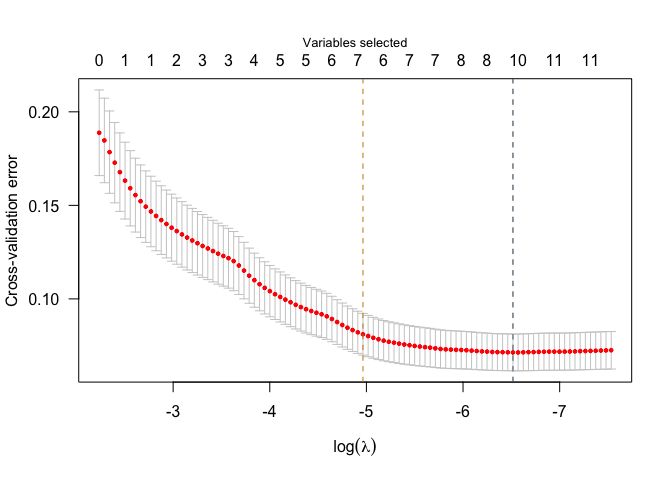``plot(srl, plot_type = "path")``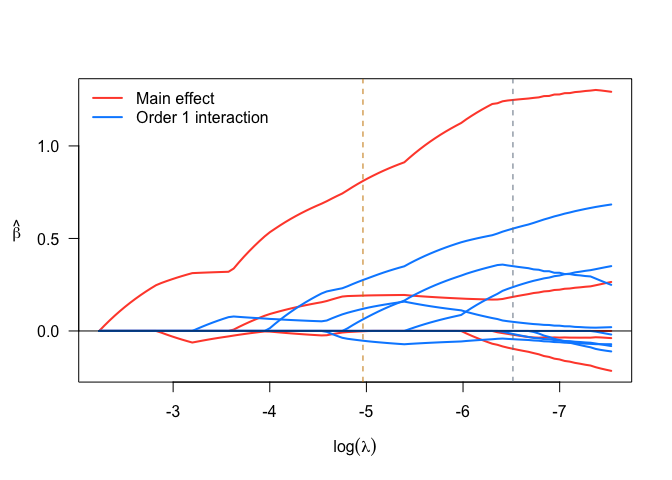Importantly, since `sparseR` with a formula centers and scales the covariates prior to forming interactions, the coefficients should be interpreted this way (a 1-unit change in the covariate corresponds to a 1 SD change in the unit of the main effect, and parameters involving interactions must be interpreted at the mean value of involved covariates).

## 2.2 Using model matrix specification

Since the `sparseR` preprocessing has limits to its customizability, and often it will be of interest to create interactions that are centered at prespecified values (as opposed to their mean), it is also possible to feed in a matrix to `sparseR` that has already been preprocessed. The function also allows users to circumvent the recipes functionality and supply their own model matrix, composed of interactions and/or polynomials. This may be useful in cases where users wish to:

• specify their own missing data imputation methods,
• specify the intercept differently than a grand mean (avoid centering variables prior to making certain interactions of interest)
• scale variables differently

The key arguments for this purpose are to set `pre_process` to `FALSE`, and supply `model_matrix` and the outcome `y`. Users may also want to specify how the model matrix is specified in terms of polynomial order (the package requires a prefix, such as “poly_1”, or “poly_2” to denote polynomial terms of orders 1 and 2 respectively) and interaction seperator (by default, this is “\\:”, which is the default for `model.matrix`).

The code below creates a model matrix will all pairwise interactions, however as opposed to the formula, the numeric variables are not centered prior to forming the interactions, and the factor variable of species will be given a reference category (as opposed to a cell-means specification). Therefore, this method will lead to different results.

``X <- model.matrix(Sepal.Width ~ .*., data = iris)[,-1]``
``````set.seed(1)
srl2 <- sparseR(pre_process = FALSE, model_matrix = X, y = iris\$Sepal.Width)``````
``## Warning in ncvreg(X = X, y = y, ...): Maximum number of iterations reached``

Since we have a warning about iterations, note that we can pass through arguments to `ncvreg` and fix this issue:

``````set.seed(1)
srl2 <- sparseR(pre_process = FALSE, model_matrix = X, y = iris\$Sepal.Width, max.iter = 1e6)``````

``srl2``
``````##
## Model summary @ min CV:
## -----------------------------------------------------
##   lasso-penalized linear regression with n=150, p=14
##   (At lambda=0.0005):
##     Nonzero coefficients: 8
##     Cross-validation error (deviance): 0.07
##     R-squared: 0.64
##     Signal-to-noise ratio: 1.77
##     Scale estimate (sigma): 0.261
##
##   SR information:
##              Vartype Total Selected Saturation Penalty
##          Main effect     5        3      0.600    2.24
##  Order 1 interaction     9        5      0.556    3.00
##
##
## Model summary @ CV1se:
## -----------------------------------------------------
##   lasso-penalized linear regression with n=150, p=14
##   (At lambda=0.0021):
##     Nonzero coefficients: 5
##     Cross-validation error (deviance): 0.08
##     R-squared: 0.59
##     Signal-to-noise ratio: 1.46
##     Scale estimate (sigma): 0.277
##
##   SR information:
##              Vartype Total Selected Saturation Penalty
##          Main effect     5        4      0.800    2.24
##  Order 1 interaction     9        1      0.111    3.00``````
``summary(srl2, at = "cv1se")``
``````## lasso-penalized linear regression with n=150, p=14
## At lambda=0.0021:
## -------------------------------------------------
##   Nonzero coefficients         :   5
##   Expected nonzero coefficients:   0.00
##   Average mfdr (5 features)    :   0.000
##
##                           Estimate      z    mfdr Selected
## Speciesvirginica           -1.1502 -25.15 < 1e-04        *
## Speciesversicolor          -1.0558 -23.10 < 1e-04        *
## Sepal.Length                0.4243  16.32 < 1e-04        *
## Petal.Width                 0.3390  12.06 < 1e-04        *
## Sepal.Length:Petal.Length  -0.0189 -11.68 < 1e-04        *``````
``plot(srl2)``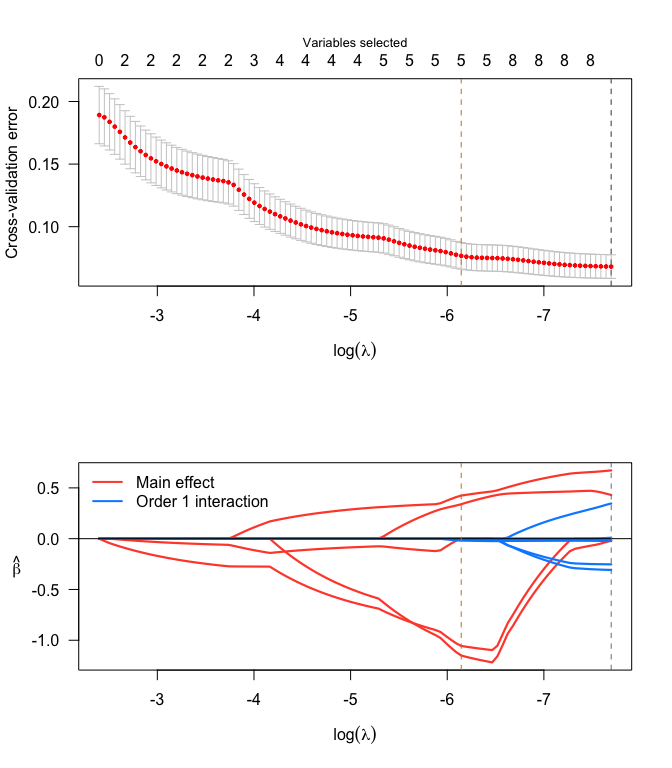Since we did not center/scale any of the variables prior to this fitting procedure, the interpretation of these coefficients should be on the original scale of the predictors, and the origin for the terms involved in interactions is 0, not at the mean of their constituent covariates.

Note also that there are fewer total covariates listed. This is because `model.matrix` uses reference cell dummy variables by default. Another way to do this without the reference category specification would be to pass pre-process options to `sparseR`, or to manually use `sparseR_prep` to create the model matrix.

``````set.seed(1)
srl3 <- sparseR(Sepal.Width ~ ., data = iris, k = 1,
pre_proc_opts = c("none"), max.iter = 1e6)
srl3``````
``````##
## Model summary @ min CV:
## -----------------------------------------------------
##   lasso-penalized linear regression with n=150, p=18
##   (At lambda=0.0007):
##     Nonzero coefficients: 6
##     Cross-validation error (deviance): 0.07
##     R-squared: 0.64
##     Signal-to-noise ratio: 1.74
##     Scale estimate (sigma): 0.262
##
##   SR information:
##              Vartype Total Selected Saturation Penalty
##          Main effect     6        2      0.333    2.45
##  Order 1 interaction    12        4      0.333    3.46
##
##
## Model summary @ CV1se:
## -----------------------------------------------------
##   lasso-penalized linear regression with n=150, p=18
##   (At lambda=0.0041):
##     Nonzero coefficients: 5
##     Cross-validation error (deviance): 0.08
##     R-squared: 0.59
##     Signal-to-noise ratio: 1.42
##     Scale estimate (sigma): 0.279
##
##   SR information:
##              Vartype Total Selected Saturation Penalty
##          Main effect     6        3      0.500    2.45
##  Order 1 interaction    12        2      0.167    3.46``````
``summary(srl3, at = "cv1se")``
``````## lasso-penalized linear regression with n=150, p=18
## At lambda=0.0041:
## -------------------------------------------------
##   Nonzero coefficients         :   5
##   Expected nonzero coefficients:   1.95
##   Average mfdr (5 features)    :   0.390
##
##                              Estimate       z    mfdr Selected
## Sepal.Length:Species_setosa  0.211340 23.4596 < 1e-04        *
## Sepal.Length                 0.233045  9.2221 < 1e-04        *
## Petal.Width                  0.179185  6.6622 < 1e-04        *
## Species_virginica           -0.056953 -1.6796 0.96137        *
## Petal.Width:Species_setosa   0.002791  0.6622 0.98794        *``````

## 2.3 Centering to different values

In many cases with interactions, it makes sense to center variables to certain values (or rather, it does not make sense to center variables to their mean or to zero). Therefore we built some flexibility into `sparseR` and `sparseR_prep` in order to allow users to change these as the context dictates.

Each covariate’s centering location (in this case their minima) can be passed into `sparseR_prep` as follows:

``````cc <- iris %>%
select(Sepal.Length, Petal.Length, Petal.Width) %>%
apply(2, min, na.rm = TRUE)

p1 <- sparseR_prep(Sepal.Width ~ ., iris, k = 0, extra_opts = list(centers = cc))
(c2min <- bake(p1, iris))``````
``````## # A tibble: 150 × 6
##    Sepal.Length Petal.Length Petal.Width Species_setosa Species_versic…¹ Speci…²
##           <dbl>        <dbl>       <dbl>          <dbl>            <dbl>   <dbl>
##  1        0.966        0.227       0.131              1                0       0
##  2        0.725        0.227       0.131              1                0       0
##  3        0.483        0.170       0.131              1                0       0
##  4        0.362        0.283       0.131              1                0       0
##  5        0.845        0.227       0.131              1                0       0
##  6        1.33         0.397       0.394              1                0       0
##  7        0.362        0.227       0.262              1                0       0
##  8        0.845        0.283       0.131              1                0       0
##  9        0.121        0.227       0.131              1                0       0
## 10        0.725        0.283       0                  1                0       0
## # … with 140 more rows, and abbreviated variable names ¹​Species_versicolor,
## #   ²​Species_virginica``````
``summary(c2min)``
``````##   Sepal.Length     Petal.Length     Petal.Width     Species_setosa
##  Min.   :0.0000   Min.   :0.0000   Min.   :0.0000   Min.   :0.0000
##  1st Qu.:0.9661   1st Qu.:0.3399   1st Qu.:0.2624   1st Qu.:0.0000
##  Median :1.8115   Median :1.8977   Median :1.5743   Median :0.0000
##  Mean   :1.8638   Mean   :1.5623   Mean   :1.4422   Mean   :0.3333
##  3rd Qu.:2.5360   3rd Qu.:2.3226   3rd Qu.:2.2303   3rd Qu.:1.0000
##  Max.   :4.3475   Max.   :3.3422   Max.   :3.1486   Max.   :1.0000
##  Species_versicolor Species_virginica
##  Min.   :0.0000     Min.   :0.0000
##  1st Qu.:0.0000     1st Qu.:0.0000
##  Median :0.0000     Median :0.0000
##  Mean   :0.3333     Mean   :0.3333
##  3rd Qu.:1.0000     3rd Qu.:1.0000
##  Max.   :1.0000     Max.   :1.0000``````

Or directly into `sparseR`:

``srl_centered2min <- sparseR(Sepal.Width ~ ., iris, extra_opts = list(centers = cc), seed = 1)``

Or, a function can be passed to evaluate the location for each covariate:

``````p2 <- sparseR_prep(Sepal.Width ~ ., iris, k = 0, extra_opts = list(center_fn = min))
(c2min2 <- bake(p2, iris))``````
``````## # A tibble: 150 × 6
##    Sepal.Length Petal.Length Petal.Width Species_setosa Species_versic…¹ Speci…²
##           <dbl>        <dbl>       <dbl>          <dbl>            <dbl>   <dbl>
##  1        0.966        0.227       0.131              1                0       0
##  2        0.725        0.227       0.131              1                0       0
##  3        0.483        0.170       0.131              1                0       0
##  4        0.362        0.283       0.131              1                0       0
##  5        0.845        0.227       0.131              1                0       0
##  6        1.33         0.397       0.394              1                0       0
##  7        0.362        0.227       0.262              1                0       0
##  8        0.845        0.283       0.131              1                0       0
##  9        0.121        0.227       0.131              1                0       0
## 10        0.725        0.283       0                  1                0       0
## # … with 140 more rows, and abbreviated variable names ¹​Species_versicolor,
## #   ²​Species_virginica``````
``identical(c2min2, c2min)``
``##  TRUE``

## 2.4 Plotting the model

Interactions are notoriously difficult to understand and to communicate, and visualizations can often be helpful. The `sparseR` package has some limited functionality for plotting the results from these fits, using the `visreg` package as a rough guide.

``effect_plot(srl, "Petal.Width", by = "Species")``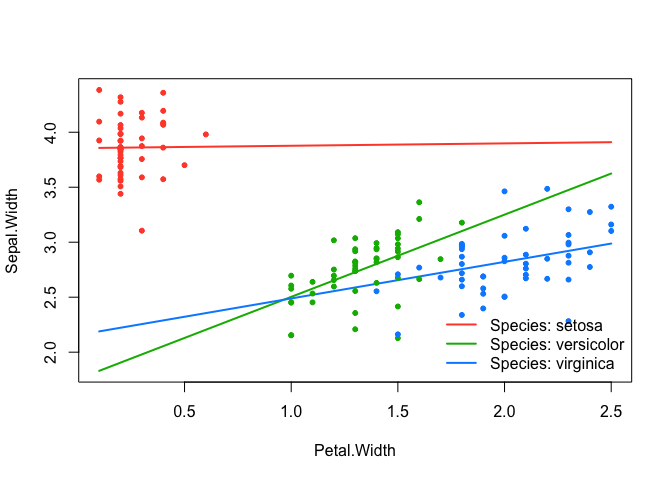Notice how this changes if the covariates are centered to their minima instead:

``effect_plot(srl_centered2min, "Petal.Width", by = "Species")``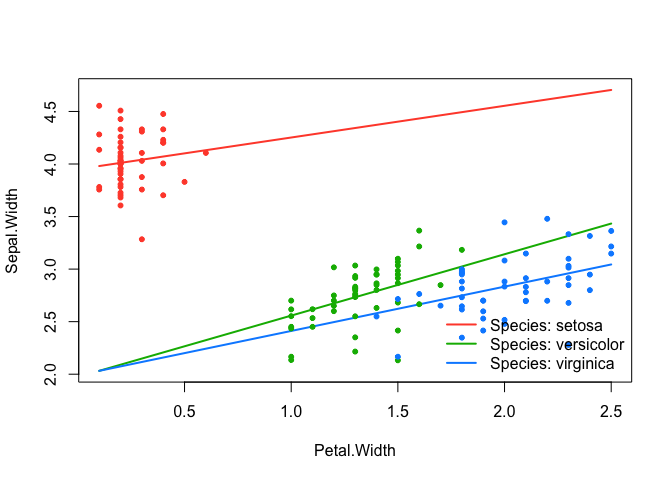It’s clear that the model itself can change quite a bit depending on the center location utilized prior to building the interactions. Unfortunately, optimizing the center location for each covariate is a difficult, p-dimensional problem (set in the context of another difficult optimization problem of regularized regression with multiple tuning parameters).

The `sparseR` package will allow users to compare certain choices in terms of their cross-validated error, which is a decent starting point. The plot below shows how the “centered to zero” model has slightly lower cross-validated error than the best mean-centered model and the best minimum-centered model.

``````plot(srl3, plot_type = "cv", ylim = c(0,.2))
abline(h = min(srl3\$fit\$cve), col = "red")
plot(srl_centered2min, plot_type = "cv", ylim = c(0,.2))
abline(h = min(srl3\$fit\$cve), col = "red")
plot(srl, plot_type = "cv", ylim = c(0,.2))
abline(h = min(srl3\$fit\$cve), col = "red")

effect_plot(srl3, "Petal.Width", by = "Species",
plot.args = list(ylim = c(1.5, 4.8)))
effect_plot(srl_centered2min, "Petal.Width", by = "Species",
plot.args = list(ylim = c(1.5, 4.8)))
effect_plot(srl, "Petal.Width", by = "Species",
plot.args = list(ylim = c(1.5, 4.8)))``````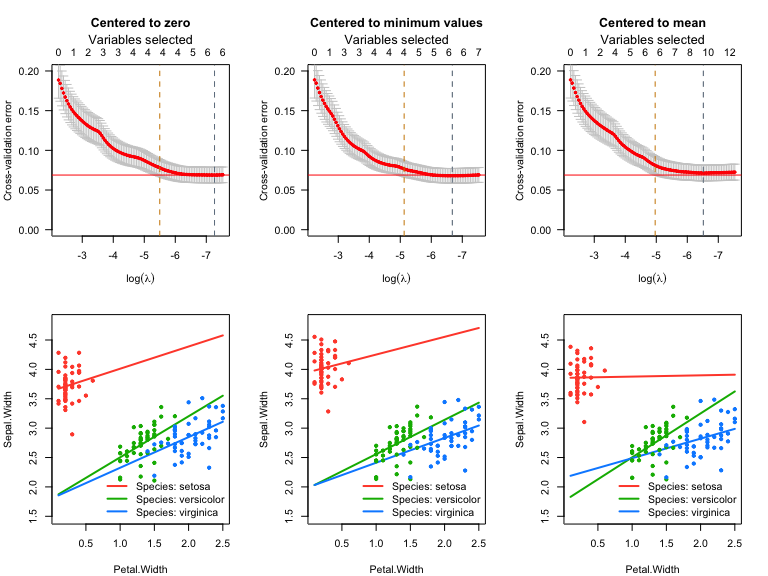Though these models look substantially different, it’s worth noting that the predictions obtained from the three models are very close to one another (since the models look similar in the neighborhood of existing data):

``````p1 <- predict(srl3, at = "cvmin")
p2 <- predict(srl_centered2min, at = "cvmin")
p3 <- predict(srl, at = "cvmin")

cor(cbind(p1, p2 ,p3))``````
``````##           p1        p2        p3
## p1 1.0000000 0.9919068 0.9919581
## p2 0.9919068 1.0000000 0.9946687
## p3 0.9919581 0.9946687 1.0000000``````
``pairs(cbind(p1, p2 ,p3))``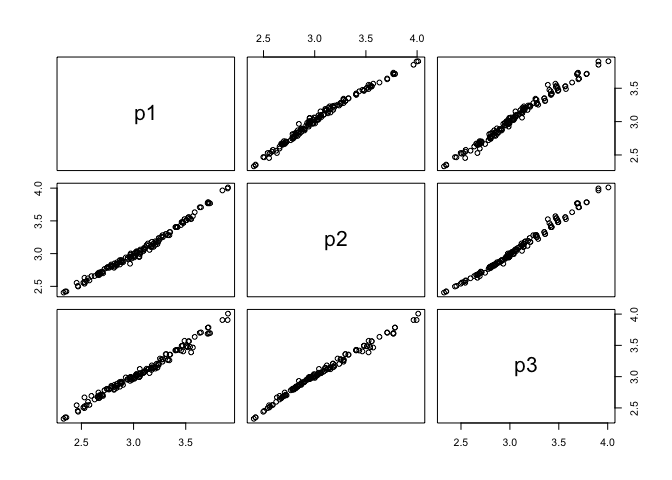At the CV1se lambda value, there is slightly less agreement in these predictions:

``````# At CV1se
p4 <- predict(srl3, at = "cv1se")
p5 <- predict(srl_centered2min, at = "cv1se")
p6 <- predict(srl, at = "cv1se")

cor(cbind(p1, p2 ,p3, p4,p5,p6))``````
``````##           p1        p2        p3        p4        p5        p6
## p1 1.0000000 0.9919068 0.9919581 0.9761025 0.9607660 0.9744660
## p2 0.9919068 1.0000000 0.9946687 0.9718119 0.9797246 0.9642979
## p3 0.9919581 0.9946687 1.0000000 0.9704648 0.9701393 0.9763341
## p4 0.9761025 0.9718119 0.9704648 1.0000000 0.9787516 0.9891270
## p5 0.9607660 0.9797246 0.9701393 0.9787516 1.0000000 0.9602063
## p6 0.9744660 0.9642979 0.9763341 0.9891270 0.9602063 1.0000000``````
``pairs(cbind(p1, p2 ,p3, p4,p5,p6))``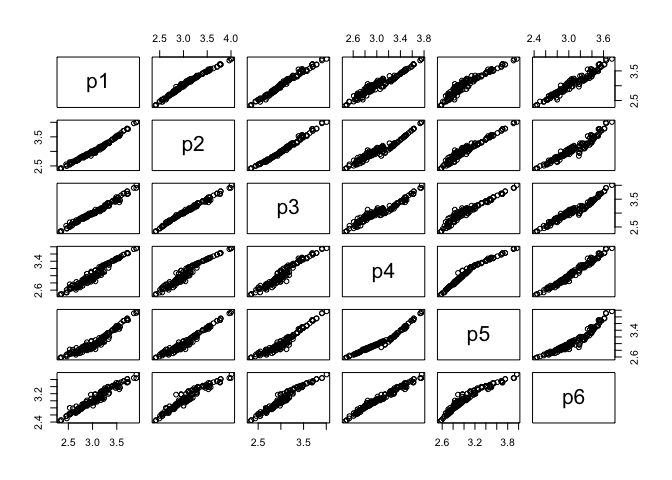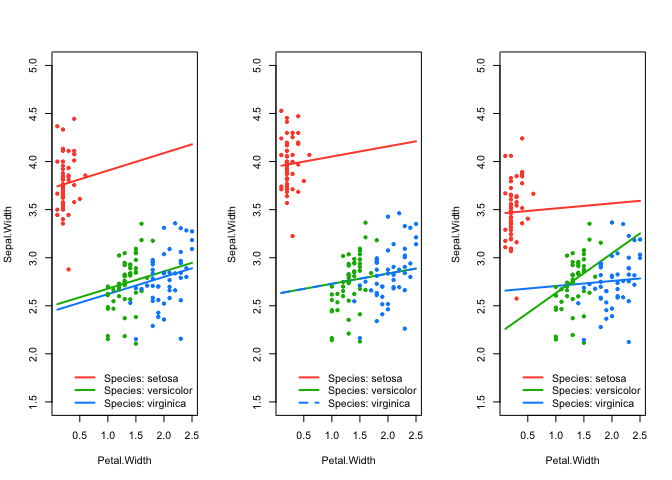``````effect_plot(srl3, "Petal.Width", by = "Species", at = "cv1se")
effect_plot(srl_centered2min, "Petal.Width", by = "Species", at = "cv1se")
effect_plot(srl, "Petal.Width", by = "Species", at = "cv1se")``````

## 2.5 Using stepwise RBIC

RBIC, or Ranked-sparsity Bayesian Information Criterion, is a ranked sparsity model selection criterion that will correctly penalize interactions (higher than main effects). The benefit of using a stepwise approach informed by RBIC is that there is no shrinkage, and it thus doesn’t matter whether or not (or where) the covariates are centered (usually). The exceptions here occur when the stepwise process is not sequential/hierarchical (i.e. main effects first, then interactions of the “active” main effects, etc.).

Inference on the parameters is still i) difficult because one must account for post-selection inference, and ii) affected by where and whether the covariates are centered. However, the lines themselves will not be affected by centering status.

We can see this in the examples below:

``````## Centered model
(rbic1 <- sparseRBIC_step(Sepal.Width ~ ., iris, pre_proc_opts = c("center", "scale")))``````
``## Note: sparseRBIC_step is currently experimental and may not behave as expected.``
``````##
## Call:  glm(formula = y ~ Species_setosa + Sepal.Length + Petal.Width +
##     Species_versicolor + Petal.Length + `Sepal.Length:Species_setosa`,
##     family = family, data = X)
##
## Coefficients:
##                   (Intercept)                 Species_setosa
##                       2.40062                        2.14130
##                  Sepal.Length                    Petal.Width
##                       0.16506                        0.44726
##            Species_versicolor                   Petal.Length
##                       0.29340                       -0.05916
## `Sepal.Length:Species_setosa`
##                       0.45943
##
## Degrees of Freedom: 149 Total (i.e. Null);  143 Residual
## Null Deviance:       28.31
## Residual Deviance: 8.999     AIC: 19.65``````
``````# Non-centered model
(rbic2 <- sparseRBIC_step(Sepal.Width ~ ., iris, pre_proc_opts = c("scale")))``````
``## Note: sparseRBIC_step is currently experimental and may not behave as expected.``
``````##
## Call:  glm(formula = y ~ Species_setosa + Sepal.Length + Petal.Width +
##     Species_versicolor + Petal.Length + `Sepal.Length:Species_setosa`,
##     family = family, data = X)
##
## Coefficients:
##                   (Intercept)                 Species_setosa
##                       0.65809                       -1.10073
##                  Sepal.Length                    Petal.Width
##                       0.16506                        0.44726
##            Species_versicolor                   Petal.Length
##                       0.29340                       -0.05916
## `Sepal.Length:Species_setosa`
##                       0.45943
##
## Degrees of Freedom: 149 Total (i.e. Null);  143 Residual
## Null Deviance:       28.31
## Residual Deviance: 8.999     AIC: 19.65``````
``````effect_plot(rbic1, "Petal.Width", by = "Species", plot.args = list(ylim = c(1.5, 5)))
effect_plot(rbic2, "Petal.Width", by = "Species", plot.args = list(ylim = c(1.5, 5)))
effect_plot(rbic1, "Sepal.Length", by = "Species")
effect_plot(rbic2, "Sepal.Length", by = "Species")``````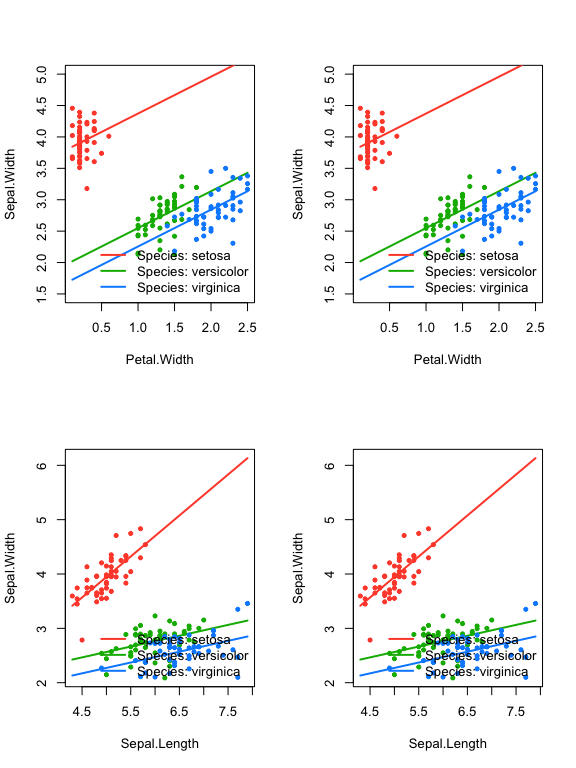Again similar S3 methods are available (note how inference does change for certain parameters based on the centering status). A message is produced because these p-values do not account for post-selection inference.

``summary(rbic1)``
``````## P-values have **not** been corrected for multiple comparisons
## Consider sparseRBIC_sampsplit() or sparseRBIC_bootstrap()``````
``````##
## Call:
## glm(formula = y ~ Species_setosa + Sepal.Length + Petal.Width +
##     Species_versicolor + Petal.Length + `Sepal.Length:Species_setosa`,
##     family = family, data = X)
##
## Deviance Residuals:
##      Min        1Q    Median        3Q       Max
## -0.78351  -0.15819   0.01874   0.16809   0.61264
##
## Coefficients:
##                               Estimate Std. Error t value Pr(>|t|)
## (Intercept)                    2.40062    0.12025  19.964  < 2e-16 ***
## Species_setosa                 2.14130    0.30584   7.001 9.10e-11 ***
## Sepal.Length                   0.16506    0.06017   2.743  0.00687 **
## Petal.Width                    0.44726    0.08833   5.064 1.25e-06 ***
## Species_versicolor             0.29340    0.09839   2.982  0.00337 **
## Petal.Length                  -0.05916    0.15021  -0.394  0.69428
## `Sepal.Length:Species_setosa`  0.45943    0.09997   4.596 9.40e-06 ***
## ---
## Signif. codes:  0 '***' 0.001 '**' 0.01 '*' 0.05 '.' 0.1 ' ' 1
##
## (Dispersion parameter for gaussian family taken to be 0.06292687)
##
##     Null deviance: 28.3069  on 149  degrees of freedom
## Residual deviance:  8.9985  on 143  degrees of freedom
## AIC: 19.646
##
## Number of Fisher Scoring iterations: 2``````
``summary(rbic2)``
``````## P-values have **not** been corrected for multiple comparisons
## Consider sparseRBIC_sampsplit() or sparseRBIC_bootstrap()``````
``````##
## Call:
## glm(formula = y ~ Species_setosa + Sepal.Length + Petal.Width +
##     Species_versicolor + Petal.Length + `Sepal.Length:Species_setosa`,
##     family = family, data = X)
##
## Deviance Residuals:
##      Min        1Q    Median        3Q       Max
## -0.78351  -0.15819   0.01874   0.16809   0.61264
##
## Coefficients:
##                               Estimate Std. Error t value Pr(>|t|)
## (Intercept)                    0.65809    0.31114   2.115  0.03616 *
## Species_setosa                -1.10073    0.60258  -1.827  0.06983 .
## Sepal.Length                   0.16506    0.06017   2.743  0.00687 **
## Petal.Width                    0.44726    0.08833   5.064 1.25e-06 ***
## Species_versicolor             0.29340    0.09839   2.982  0.00337 **
## Petal.Length                  -0.05916    0.15021  -0.394  0.69428
## `Sepal.Length:Species_setosa`  0.45943    0.09997   4.596 9.40e-06 ***
## ---
## Signif. codes:  0 '***' 0.001 '**' 0.01 '*' 0.05 '.' 0.1 ' ' 1
##
## (Dispersion parameter for gaussian family taken to be 0.06292687)
##
##     Null deviance: 28.3069  on 149  degrees of freedom
## Residual deviance:  8.9985  on 143  degrees of freedom
## AIC: 19.646
##
## Number of Fisher Scoring iterations: 2``````

Alternatively, sample splitting can be utilized to achieve valid inference. This method divides the data into two samples of equal size, uses the first sample to select the model, and the second one to fit and achieve valid (though potentially low-power) inference. Coefficients which are not selected are bestowed a p-value of 1.

``s1 <- sparseRBIC_sampsplit(rbic1)``
``## Note: sparseRBIC_sampsplit is currently experimental and may not behave as expected.``
``````## Warning: `progress_estimated()` was deprecated in dplyr 1.0.0.
## ℹ The deprecated feature was likely used in the sparseR package.
##   Please report the issue to the authors.``````
Coefficient Median_p Mean_p Gmean_p % selected
(Intercept) 0.00000 0.00000 0.00000 100
Species_setosa 0.00000 0.00000 0.00000 100
Sepal.Length 0.00028 0.11109 0.00012 90
Sepal.Length:Species_setosa 0.00284 0.20263 0.00524 80
Petal.Width 1.00000 0.60319 0.09233 40
Species_versicolor 1.00000 0.70857 0.24735 30
Petal.Length 1.00000 0.96673 0.96036 10
``s2 <- sparseRBIC_sampsplit(rbic2)``
``## Note: sparseRBIC_sampsplit is currently experimental and may not behave as expected.``
Coefficient Median_p Mean_p Gmean_p % selected
(Intercept) 0.04286 0.24330 0.02898 100
Species_setosa 0.09819 0.16317 0.00690 100
Sepal.Length 0.00063 0.00644 0.00008 100
Petal.Width 0.06679 0.41560 0.06462 60
Species_virginica 1.00000 0.71881 0.38100 30
Sepal.Length:Species_setosa 0.01678 0.20926 0.00805 80
Species_versicolor 1.00000 0.81515 0.57679 20
Petal.Width:Species_setosa 1.00000 0.92315 0.90718 20
Petal.Length 1.00000 0.98405 0.98277 10
Sepal.Length:Petal.Length 1.00000 0.93700 0.90536 10

Additionally, inferences based on bootstrapped samples of the data are available via the `sparseRBIC_bootstrap` function, which stores p-values for each coefficient after model selection has been performed. If a coefficient has not been selected, the p-value is set to one for that bootstrap iteration, so when the “mean” p-value is calculated across bootstraps, the underestimation of the p-value due to model selection is attenuated, as the estimate gets “pulled up” by the 1’s in the vector. Since this may be a conservative approach, we also display the “geometric” mean which is a measure of central tendency less sensitive to skew (which many p-values will be highly right skewed). More research must be done on these techniques to ensure adequate coverage properties, but given a high enough number of bootstrap samples they should both be more conservative than the naive, “pretend model selection never took place” approach. The downside here is that depending on the size of the data and the number of variables, this could take quite some time.

Note that the mean p-value across bootstraps is bounded to be greater than 1-P(selected). In some sense, this makes sense, as if a variable has a low probability of being selected, then that variable should have a higher adjusted p-value. However, for variables whose effects will be highly related (i.e. an interaction and a constituent main effect), this may be too conservative.

``````set.seed(1)
## Centered model
b1 <- sparseRBIC_bootstrap(rbic1)``````
Coefficient Mean_p Gmean_p % selected
(Intercept) 0.00000 0.00000 100
Species_setosa 0.00000 0.00000 100
Sepal.Length 0.00293 0.00000 100
Sepal.Length:Species_setosa 0.20011 0.00002 80
Petal.Width 0.50000 0.00008 50
Species_versicolor 0.60010 0.01626 40
Petal.Length 0.81087 0.54467 20
Sepal.Length:Petal.Width 0.90000 0.32270 10
Sepal.Length:Species_versicolor 0.90222 0.68331 10
Petal.Width:Species_setosa 0.90182 0.66977 10
Sepal.Length:Petal.Length 0.90000 0.22450 10
``````set.seed(1)
## Uncentered model
b2 <- sparseRBIC_bootstrap(rbic2)``````
``## Note: sparseRBIC_bootstrap is currently experimental and may not behave as expected.``
Coefficient Mean_p Gmean_p % selected
(Intercept) 0.16411 0.00016 100
Species_setosa 0.08507 0.00002 100
Sepal.Length 0.00293 0.00000 100
Sepal.Length:Species_setosa 0.20011 0.00002 80
Petal.Width 0.50000 0.00017 50
Species_versicolor 0.60079 0.02124 40
Petal.Length 0.80312 0.38407 20
Sepal.Length:Petal.Width 0.90000 0.32270 10
Sepal.Length:Species_versicolor 0.90222 0.68331 10
Petal.Width:Species_setosa 0.90182 0.66977 10
Sepal.Length:Petal.Length 0.90000 0.22450 10

This section is still under development.

# 3 Use case: lung data

As a more realistic example, we take a data set that is often used to train models that can predict lung cancer status. It has 1027 observations and 14 covariates, including urban/rural, age, years of school, smoking years, number of children, etc.

First, let’s look at the data a little:

``````data("irlcs_radon_syn")
``````##        ID         CITY       YRSHOME          AGE        CASE    SCHOOL
##  Min.   :   1.0   0:246   Min.   :20.0   Min.   :44.16   0:632   1: 92
##  1st Qu.: 260.5   1:781   1st Qu.:25.0   1st Qu.:61.38   1:395   2:506
##  Median : 492.0           Median :31.0   Median :67.84           3:293
##  Mean   : 505.2           Mean   :33.1   Mean   :67.45           4:106
##  3rd Qu.: 761.0           3rd Qu.:39.0   3rd Qu.:73.82           5: 30
##  Max.   :1026.0           Max.   :75.0   Max.   :84.80
##
##      SMKYRS         CHILDREN           PYR            PYRRATE
##  Min.   : 0.00   Min.   : 0.000   Min.   :  0.00   Min.   :0.00000
##  1st Qu.: 0.00   1st Qu.: 2.000   1st Qu.:  0.00   1st Qu.:0.00000
##  Median :13.00   Median : 3.000   Median :  3.60   Median :0.05368
##  Mean   :20.58   Mean   : 3.065   Mean   : 19.31   Mean   :0.31745
##  3rd Qu.:41.00   3rd Qu.: 4.000   3rd Qu.: 35.07   3rd Qu.:0.58024
##  Max.   :64.00   Max.   :13.000   Max.   :138.45   Max.   :2.55702
##
##     SMKQUIT            BMI            WLM20        PRELUNG SMKEVER SMKCUR
##  Min.   : 0.000   Min.   :16.64   Min.   : 2.176   0:692   0:476   0:705
##  1st Qu.: 0.000   1st Qu.:21.79   1st Qu.: 5.902   1:335   1:551   1:322
##  Median : 0.000   Median :23.78   Median : 8.683
##  Mean   : 4.733   Mean   :24.60   Mean   :10.914
##  3rd Qu.: 3.325   3rd Qu.:27.40   3rd Qu.:13.246
##  Max.   :57.355   Max.   :41.60   Max.   :77.457
##                   NA's   :51``````

Note:

• There are missing values for BMI - we will see how `sparseR` handles this.
• Everything is coded as numeric except the outcome, case (this is not necessary for `sparseR` to work), as we will see.
• `ID` is an arbitrary identifying variable which should not be used in the modeling phase
``irlcs_radon_syn <- select(irlcs_radon_syn, -ID)``

At this point, we will split the data into a test and training set.

``````set.seed(13)

trainIDX <- sample(1:N, N * .75)

## 3.1 Preprocessing

Although the `sparseR()` function will do it automatically, it’s generally a good idea to see how the data is being preprocessed and to examine how the model matrix is built before the regularization. We can see what `sparseR` does to preprocess the data by running the function `sparseR_prep`, which utilizes the `recipes` package to perform preprocessing. At no time is the outcome used to train any of the steps, except for the elimination of NA values if any exist.

``````prep_obj <- sparseR_prep(CASE ~ ., data = train, k = 0, poly = 1)
prep_obj``````
``## ``
``## ── Recipe ──────────────────────────────────────────────────────────────────────``
``## ``
``## ── Inputs``
``## Number of variables by role``
``````## outcome:    1
## predictor: 10
## dummy:      4``````
``## ``
``## ── Training information``
``## Training data contained 770 data points and 43 incomplete rows.``
``## ``
``## ── Operations``
``## • Variables removed: <none> | Trained``
``## • Sparse, unbalanced variable filter removed: <none> | Trained``
``## Centering to mean for YRSHOME, AGE, SMKYRS, CHILDREN, PYR, PYRRATE, S... [trained]``
``## • Scaling for: YRSHOME, AGE, SMKYRS, CHILDREN, PYR, PYRRATE, ... | Trained``
``## • Collapsing factor levels for: SCHOOL | Trained``
``## • K-nearest neighbor imputation for: YRSHOME, AGE, SCHOOL, ... | Trained``
``## • Removing rows with NA values in: CITY, YRSHOME, AGE, SCHOOL, ... | Trained``
``## • Variables removed: CASE | Trained``
``## • Dummy variables from: CITY, SCHOOL, PRELUNG, SMKEVER, SMKCUR | Trained``
``## • Sparse, unbalanced variable filter removed: SCHOOL_other | Trained``

We see that the preprocessor did many things, among which it imputed the data for the missing value using the other predictors in the model. Apparently, by default, this preprocessor will remove variables with “near” zero variance, and that as a result, SMKYRS is not considered (it’s eliminated in an early step).

If we look at this variable, we see that it may be informative:

``MASS::truehist(train\$SMKYRS)``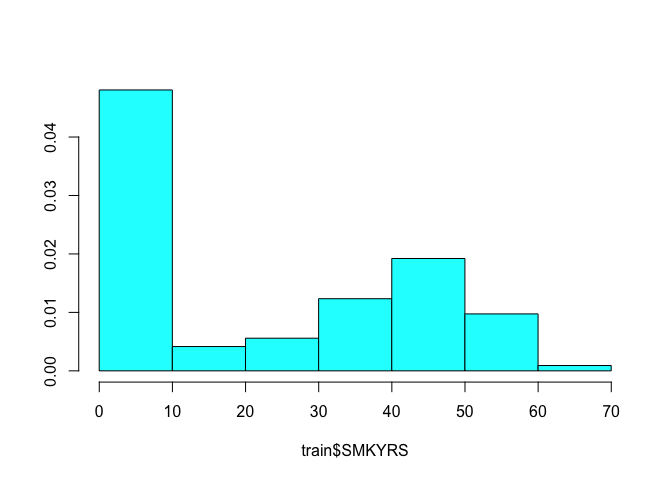Therefore, we can tell the preprocessor not to filter this variable in two ways. First, we could tell it to use `zv` instead of `nzv`, in which case it will only remove zero variance predictors:

``sparseR_prep(CASE ~ ., data = train, k = 0, poly = 1, filter = "zv")``
``## ``
``## ── Recipe ──────────────────────────────────────────────────────────────────────``
``## ``
``## ── Inputs``
``## Number of variables by role``
``````## outcome:    1
## predictor: 10
## dummy:      4``````
``## ``
``## ── Training information``
``## Training data contained 770 data points and 43 incomplete rows.``
``## ``
``## ── Operations``
``## • Variables removed: <none> | Trained``
``## • Zero variance filter removed: <none> | Trained``
``## Centering to mean for YRSHOME, AGE, SMKYRS, CHILDREN, PYR, PYRRATE, S... [trained]``
``## • Scaling for: YRSHOME, AGE, SMKYRS, CHILDREN, PYR, PYRRATE, ... | Trained``
``## • Collapsing factor levels for: SCHOOL | Trained``
``## • K-nearest neighbor imputation for: YRSHOME, AGE, SCHOOL, ... | Trained``
``## • Removing rows with NA values in: CITY, YRSHOME, AGE, SCHOOL, ... | Trained``
``## • Variables removed: CASE | Trained``
``## • Dummy variables from: CITY, SCHOOL, PRELUNG, SMKEVER, SMKCUR | Trained``
``## • Zero variance filter removed: <none> | Trained``

Or, we could adjust the options for the near-zero variance filter (see `?recipes::step_nzv` for details):

``sparseR_prep(CASE ~ ., data = train, k = 0, poly = 1, extra_opts = list(unique_cut = 5))``
``## ``
``## ── Recipe ──────────────────────────────────────────────────────────────────────``
``## ``
``## ── Inputs``
``## Number of variables by role``
``````## outcome:    1
## predictor: 10
## dummy:      4``````
``## ``
``## ── Training information``
``## Training data contained 770 data points and 43 incomplete rows.``
``## ``
``## ── Operations``
``## • Variables removed: <none> | Trained``
``## • Sparse, unbalanced variable filter removed: <none> | Trained``
``## Centering to mean for YRSHOME, AGE, SMKYRS, CHILDREN, PYR, PYRRATE, S... [trained]``
``## • Scaling for: YRSHOME, AGE, SMKYRS, CHILDREN, PYR, PYRRATE, ... | Trained``
``## • Collapsing factor levels for: SCHOOL | Trained``
``## • K-nearest neighbor imputation for: YRSHOME, AGE, SCHOOL, ... | Trained``
``## • Removing rows with NA values in: CITY, YRSHOME, AGE, SCHOOL, ... | Trained``
``## • Variables removed: CASE | Trained``
``## • Dummy variables from: CITY, SCHOOL, PRELUNG, SMKEVER, SMKCUR | Trained``
``## • Sparse, unbalanced variable filter removed: SCHOOL_other | Trained``

Notice that we haven’t told the preprocessor to build any interactions or polynomials of higher degree than 1. we can do this simply by setting `k` and `poly` respectively to higher numbers:

``sparseR_prep(CASE ~ ., data = train, k = 1, poly = 1, extra_opts = list(unique_cut = 5))``
``## ``
``## ── Recipe ──────────────────────────────────────────────────────────────────────``
``## ``
``## ── Inputs``
``## Number of variables by role``
``````## outcome:    1
## predictor: 10
## dummy:      4``````
``## ``
``## ── Training information``
``## Training data contained 770 data points and 43 incomplete rows.``
``## ``
``## ── Operations``
``## • Variables removed: <none> | Trained``
``## • Sparse, unbalanced variable filter removed: <none> | Trained``
``## Centering to mean for YRSHOME, AGE, SMKYRS, CHILDREN, PYR, PYRRATE, S... [trained]``
``## • Scaling for: YRSHOME, AGE, SMKYRS, CHILDREN, PYR, PYRRATE, ... | Trained``
``## • Collapsing factor levels for: SCHOOL | Trained``
``## • K-nearest neighbor imputation for: YRSHOME, AGE, SCHOOL, ... | Trained``
``## • Removing rows with NA values in: CITY, YRSHOME, AGE, SCHOOL, ... | Trained``
``## • Variables removed: CASE | Trained``
``## • Dummy variables from: CITY, SCHOOL, PRELUNG, SMKEVER, SMKCUR | Trained``
``````## • Interactions with: (YRSHOME + AGE + SMKYRS + CHILDREN + PYR + PYRRATE +
##   SMKQUIT + BMI + WLM20 + (CITY_X0 + CITY_X1 + SCHOOL_X1 + SCHOOL_X2 +
##   SCHOOL_X3 + SCHOOL_X4 + SCHOOL_other + PRELUNG_X0 + PRELUNG_X1 + SMKEVER_X0 +
##   SMKEVER_X1 + SMKCUR_X0 + SMKCUR_X1)) * (YRSHOME + AGE + SMKYRS + CHILDREN +
##   PYR + PYRRATE + SMKQUIT + BMI + WLM20 + (CITY_X0 + CITY_X1 + SCHOOL_X1 +
##   SCHOOL_X2 + SCHOOL_X3 + SCHOOL_X4 + SCHOOL_other + PRELUNG_X0 + PRELUNG_X1 +
##   SMKEVER_X0 + SMKEVER_X1 + SMKCUR_X0 + SMKCUR_X1)) | Trained``````
``## • Sparse, unbalanced variable filter removed: SCHOOL_other, ... | Trained``
``sparseR_prep(CASE ~ ., data = train, k = 1, poly = 2, extra_opts = list(unique_cut = 5))``
``## ``
``## ── Recipe ──────────────────────────────────────────────────────────────────────``
``## ``
``## ── Inputs``
``## Number of variables by role``
``````## outcome:    1
## predictor: 10
## dummy:      4``````
``## ``
``## ── Training information``
``## Training data contained 770 data points and 43 incomplete rows.``
``## ``
``## ── Operations``
``## • Variables removed: <none> | Trained``
``## • Sparse, unbalanced variable filter removed: <none> | Trained``
``## Centering to mean for YRSHOME, AGE, SMKYRS, CHILDREN, PYR, PYRRATE, S... [trained]``
``## • Scaling for: YRSHOME, AGE, SMKYRS, CHILDREN, PYR, PYRRATE, ... | Trained``
``## • Collapsing factor levels for: SCHOOL | Trained``
``## • K-nearest neighbor imputation for: YRSHOME, AGE, SCHOOL, ... | Trained``
``## • Removing rows with NA values in: CITY, YRSHOME, AGE, SCHOOL, ... | Trained``
``## • Variables removed: CASE | Trained``
``## • Dummy variables from: CITY, SCHOOL, PRELUNG, SMKEVER, SMKCUR | Trained``
``````## • Interactions with: (YRSHOME + AGE + SMKYRS + CHILDREN + PYR + PYRRATE +
##   SMKQUIT + BMI + WLM20 + (CITY_X0 + CITY_X1 + SCHOOL_X1 + SCHOOL_X2 +
##   SCHOOL_X3 + SCHOOL_X4 + SCHOOL_other + PRELUNG_X0 + PRELUNG_X1 + SMKEVER_X0 +
##   SMKEVER_X1 + SMKCUR_X0 + SMKCUR_X1)) * (YRSHOME + AGE + SMKYRS + CHILDREN +
##   PYR + PYRRATE + SMKQUIT + BMI + WLM20 + (CITY_X0 + CITY_X1 + SCHOOL_X1 +
##   SCHOOL_X2 + SCHOOL_X3 + SCHOOL_X4 + SCHOOL_other + PRELUNG_X0 + PRELUNG_X1 +
##   SMKEVER_X0 + SMKEVER_X1 + SMKCUR_X0 + SMKCUR_X1)) | Trained``````
``## • Orthogonal polynomials on: YRSHOME, AGE, SMKYRS, CHILDREN, PYR, ... | Trained``
``## • Sparse, unbalanced variable filter removed: SCHOOL_other, ... | Trained``

Note: This preprocessor does not actually produce any data, it only prepares all of the steps. In the recipes package, this is known as `prep`ping the processor. the final step is called `bake`, where the data is fed through the processor and a new data set is returned.

## 3.2 Using the `sparseR` function

All of the preprocessing options can be accomplished through the main function for the package, `sparseR`. The following code runs a SRL model, an APL model, a main effects model, and an SRL with polynomials:

``````lso <- list(
SRL  = sparseR(CASE ~ ., train, seed = 1),            ## SRL model
APL  = sparseR(CASE ~ ., train, seed = 1, gamma = 0), ## APL model
ME   = sparseR(CASE ~ ., train, seed = 1, k = 0),     ## Main effects model
SRLp = sparseR(CASE ~ ., train, seed = 1, poly = 2)   ## SRL + polynomials
)``````

The simple print method allows one to investigate all of these models

``lso``
``````## \$SRL
##
## Model summary @ min CV:
## -----------------------------------------------------
##   lasso-penalized linear regression with n=770, p=195
##   (At lambda=0.0007):
##     Nonzero coefficients: 42
##     Cross-validation error (deviance): 0.14
##     R-squared: 0.43
##     Signal-to-noise ratio: 0.74
##     Scale estimate (sigma): 0.368
##
##   SR information:
##              Vartype Total Selected Saturation Penalty
##          Main effect    21       10      0.476    4.58
##  Order 1 interaction   174       32      0.184   13.19
##
##
## Model summary @ CV1se:
## -----------------------------------------------------
##   lasso-penalized linear regression with n=770, p=195
##   (At lambda=0.0098):
##     Nonzero coefficients: 4
##     Cross-validation error (deviance): 0.14
##     R-squared: 0.40
##     Signal-to-noise ratio: 0.65
##     Scale estimate (sigma): 0.378
##
##   SR information:
##              Vartype Total Selected Saturation Penalty
##          Main effect    21        4       0.19    4.58
##  Order 1 interaction   174        0       0.00   13.19
##
## \$APL
##
## Model summary @ min CV:
## -----------------------------------------------------
##   lasso-penalized linear regression with n=770, p=195
##   (At lambda=0.0095):
##     Nonzero coefficients: 45
##     Cross-validation error (deviance): 0.14
##     R-squared: 0.42
##     Signal-to-noise ratio: 0.72
##     Scale estimate (sigma): 0.370
##
##   SR information:
##              Vartype Total Selected Saturation Penalty
##          Main effect    21        5      0.238       1
##  Order 1 interaction   174       40      0.230       1
##
##
## Model summary @ CV1se:
## -----------------------------------------------------
##   lasso-penalized linear regression with n=770, p=195
##   (At lambda=0.0555):
##     Nonzero coefficients: 6
##     Cross-validation error (deviance): 0.14
##     R-squared: 0.39
##     Signal-to-noise ratio: 0.64
##     Scale estimate (sigma): 0.380
##
##   SR information:
##              Vartype Total Selected Saturation Penalty
##          Main effect    21        3     0.1429       1
##  Order 1 interaction   174        3     0.0172       1
##
## \$ME
##
## Model summary @ min CV:
## -----------------------------------------------------
##   lasso-penalized linear regression with n=770, p=21
##   (At lambda=0.0030):
##     Nonzero coefficients: 10
##     Cross-validation error (deviance): 0.14
##     R-squared: 0.41
##     Signal-to-noise ratio: 0.70
##     Scale estimate (sigma): 0.373
##
##   SR information:
##      Vartype Total Selected Saturation Penalty
##  Main effect    21       10      0.476    4.58
##
##
## Model summary @ CV1se:
## -----------------------------------------------------
##   lasso-penalized linear regression with n=770, p=21
##   (At lambda=0.0158):
##     Nonzero coefficients: 3
##     Cross-validation error (deviance): 0.15
##     R-squared: 0.38
##     Signal-to-noise ratio: 0.61
##     Scale estimate (sigma): 0.383
##
##   SR information:
##      Vartype Total Selected Saturation Penalty
##  Main effect    21        3      0.143    4.58
##
## \$SRLp
##
## Model summary @ min CV:
## -----------------------------------------------------
##   lasso-penalized linear regression with n=770, p=204
##   (At lambda=0.0009):
##     Nonzero coefficients: 41
##     Cross-validation error (deviance): 0.13
##     R-squared: 0.43
##     Signal-to-noise ratio: 0.75
##     Scale estimate (sigma): 0.367
##
##   SR information:
##              Vartype Total Selected Saturation Penalty
##          Main effect    21       11      0.524    4.58
##  Order 1 interaction   174       24      0.138   13.19
##   Order 2 polynomial     9        6      0.667    5.48
##
##
## Model summary @ CV1se:
## -----------------------------------------------------
##   lasso-penalized linear regression with n=770, p=204
##   (At lambda=0.0093):
##     Nonzero coefficients: 4
##     Cross-validation error (deviance): 0.14
##     R-squared: 0.40
##     Signal-to-noise ratio: 0.66
##     Scale estimate (sigma): 0.377
##
##   SR information:
##              Vartype Total Selected Saturation Penalty
##          Main effect    21        3      0.143    4.58
##  Order 1 interaction   174        0      0.000   13.19
##   Order 2 polynomial     9        1      0.111    5.48``````

Similarly, plots can be produced of all of these figures:

``n <- lapply(lso, plot, log.l = TRUE)``